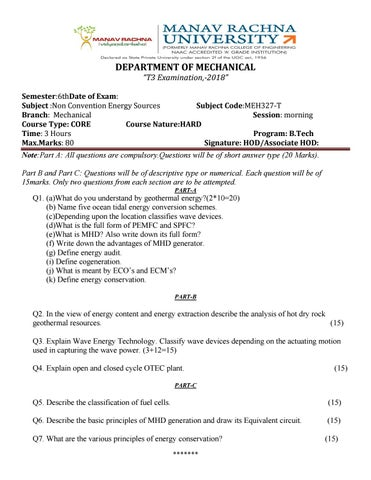DEPARTMENT OF MECHANICAL “T3 Examination,-2018” Semester:6thDate of Exam: Subject :Non Convention Energy Sources Subject Code:MEH327-T Branch: Mechanical Session: morning Course Type: CORE Course Nature:HARD Time: 3 Hours Program: B.Tech Max.Marks: 80 Signature: HOD/Associate HOD: Note:Part A: All questions are compulsory.Questions will be of short answer type (20 Marks). Part B and Part C: Questions will be of descriptive type or numerical. Each question will be of 15marks. Only two questions from each section are to be attempted. PART-A

Q1. (a)What do you understand by geothermal energy?(2*10=20) (b) Name five ocean tidal energy conversion schemes. (c)Depending upon the location classifies wave devices. (d)What is the full form of PEMFC and SPFC? (e)What is MHD? Also write down its full form? (f) Write down the advantages of MHD generator. (g) Define energy audit. (i) Define cogeneration. (j) What is meant by ECO’s and ECM’s? (k) Define energy conservation. PART-B

Q2. In the view of energy content and energy extraction describe the analysis of hot dry rock geothermal resources. (15) Q3. Explain Wave Energy Technology. Classify wave devices depending on the actuating motion used in capturing the wave power. (3+12=15) Q4. Explain open and closed cycle OTEC plant.

(15)

PART-C

Q5. Describe the classification of fuel cells.

(15)

Q6. Describe the basic principles of MHD generation and draw its Equivalent circuit.

(15)

Q7. What are the various principles of energy conservation? *******

(15)

*******